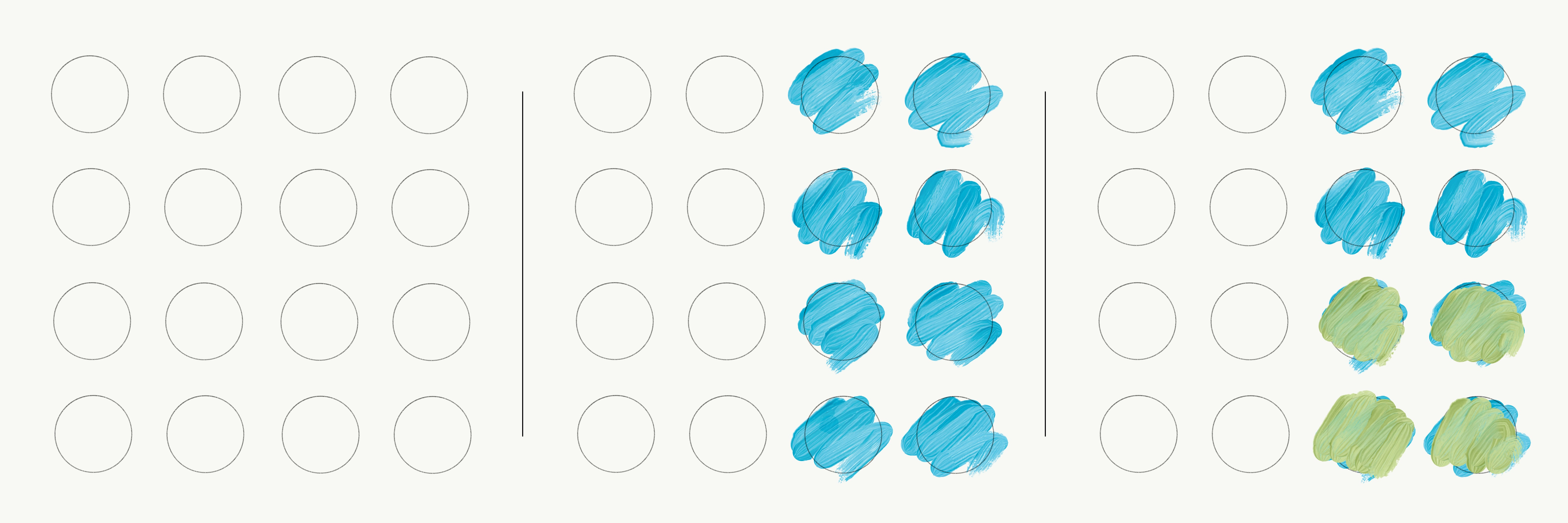# When and why do you multiply probabilities?

### At high school you may have been taught that, sometimes, you have to multiply probabilities. We briefly discuss when and why you do this.

First a few notes on the notation of probabilities. When throwing with a dice, the event of throwing a six is 1 of 6 possibilities. We write this as a fraction, 1/6, or

$\frac{1}{6}.$

We then say there is a probability of 1 out of 6 to throw, for instance, a 6. The probability is 1/6, one sixth.

This also means that the probability of throwing a number—this can thus be any number: 1, 2, 3, 4, 5 or 6—is equal to $\frac{6}{6} = 1.$

If you throw a dice, the probability is 1 for throwing a number, or 100%. In other words, if something is 100% certain to happen, the probability is 1. And if something is less certain to occur, less than 100%, the probability is an n’th part of 1.

Lastly, an important announcement on multiplying by a fraction: if you calculate an n’th part of something, for instance, 16, you can write this in two ways. You divide 16 by 2 or you multiply 16 by 1/2. It is the same. That is: $\frac{16}{2} = 16\times\frac{1}{2} = 8.$

### Two coins

Suppose, you throw euro #1 into the air. It is going to be either heads or tails. In Figure fig:figure1, this is represented schematically. The probability of throwing heads is 1/2. The odds of throwing tails is 1/2.

Imagine throwing euro #1 and euro #2 into the air. This is represented in Figure 1.

Now, ask yourself the question: of all the times I threw heads with euro #1, how many times would I have thrown euro #2? The answer is that half of the time euro #1 became heads and half of that time europ #2 became heads.

What is half of a half? This is
$\underbrace{\frac{1/2}{2}}_\text{half of a half} = \frac{1}{2} \times \frac{1}{2} = \frac{1}{4}.$

### Part of a part

See Figure fig:figure3. Suppose, we throw two coins 16 times. Suppose, coin number 1 turns out heads half the time; we signify this with blue circles. The question is how many times that coin number 1 is heads, do we throw heads with the second coin? This is, again, half. Half of half, that is. We paint this green.

Of the total amount of throws, what part is green? Half (4) of half (8) of the total (16), so 4 out of 16, or 1 out of 4. So, what is the probability of throwing green (coin number 2 is heads) if you throw blue (coin number 1 is heads) half of the time. $\frac{1/2}{2} = \frac{1}{2}\times\frac{1}{2} = \frac{1}{4}.$

Of all the trials where the euro turned out to be heads—which is half of the total amount of trials—how many times would you have thrown a 6 with the dice? That is, thus, 1/6th of one half of the total amount of trials. Or, $\frac{1/2}{6} = \frac{1}{2} \times \frac{1}{6} = \frac{1}{12}.$
Suppose, that the probability is 2/3 for event $A$ to happen, the probability is 1/6 for event $B$ to occur, and 4/5 that even $C$ will happen, then the probability of the combination of the events $A$, $B$, and $C$ to occur is equal to $\underbrace{\frac{2}{3}}_A \times \underbrace{\frac{1}{6}}_B \times \underbrace{\frac{4}{5}}_C = \frac{8}{90} = \frac{4}{45} \approx 0.088\dots,$ which is 8.9% rounded to one decimal.